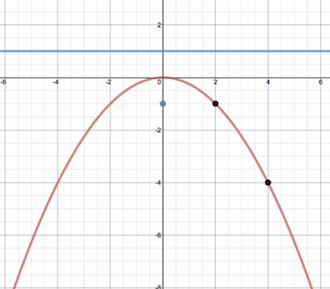# Parabolas Lesson 5 Episode 4 (Teachers)

Keoni and Sasha determine the equation of a parabola with a vertex at (0,0) and distance of three units between its focus and vertex. They compare the equation to their conjectures from Episode 3.

### Episode SupportsFocus Questions

For use in a classroom, pause the video and ask this question:

1. [Pause video at 1:58]. For the quantity y – 3, where on the graph is the distance y? 3? y – 3?

Supporting Dialogue

Provide the opportunity for involvement of all the students in the room by asking open-ended questions that can support many good observations:

1. After Sasha and Keoni have developed two forms for the equation [4:01], ask “How do you know that each equation works?” If there is not an immediate response, ask students to share ideas with a neighbor.

2. How do you know that the equations  x = √(12y) and y = x2/12 are equivalent?

Math Extensions

1. Below is a graph of a parabola with a vertex at (0,0) and a focus at (0, –1). Using the definition of a parabola, find the coordinates of the two indicated points on the parabola.

2. Explain how the coordinates of the points relate to the distances between the each point and the directrix and between the each point and the focus.